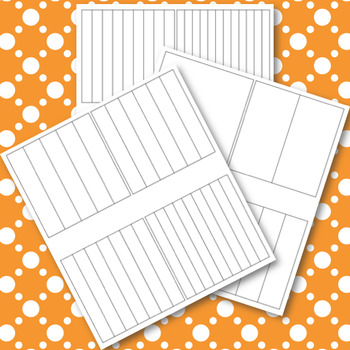# Intervention Fraction ManipulativesSubject
Resource Type
Format
PDF (11 MB)
Standards
\$2.00
\$2.00

### Description

Small Groups Instruction Number Lines and Area Models

Students can use number lines and area models to find equivalent fractions, add and subtract and estimation.

How to Use the Cards:

Print the cards on cardstock and laminate. Use in small group instruction with manipulatives to help students make a connections and increase their understanding of fractions.

Skills:

Number and operations. The student applies mathematical process standards to represent and generate fractions to solve problems. The student is expected to:

4.3(A) represent a fraction a/b as a sum of fractions 1/b, where a and b are whole numbers and b > 0, including

when a > b

4.3(B) decompose a fraction in more than one way into a sum of fractions with the same denominator using

concrete and pictorial models and recording results with symbolic representations

4.3(C) determine if two given fractions are equivalent using a variety of methods

4.3(D) compare two fractions with different numerators and different denominators and represent the comparison using the symbols >, =, or <

4.3(E) represent and solve addition and subtraction of fractions with equal denominators using objects and pictorial models that build to the number line and properties of operations

4.3(F) evaluate the reasonableness of sums and differences of fractions using benchmark fractions 0, 1/4, 1/2, 3/4, and 1, referring to the same whole

4.3(G) represent fractions and decimals to the tenths or hundredths as distances from zero on a number line

Total Pages
N/A
N/A
Teaching Duration
N/A
Report this Resource to TpT
Reported resources will be reviewed by our team. Report this resource to let us know if this resource violates TpT’s content guidelines.

### Standards

to see state-specific standards (only available in the US).
Decompose a fraction into a sum of fractions with the same denominator in more than one way, recording each decomposition by an equation. Justify decompositions, e.g., by using a visual fraction model. Examples: 3/8 = 1/8 + 1/8 + 1/8; 3/8 = 1/8 + 2/8; 2 1/8 = 1 + 1 + 1/8 = 8/8 + 8/8 + 1/8.